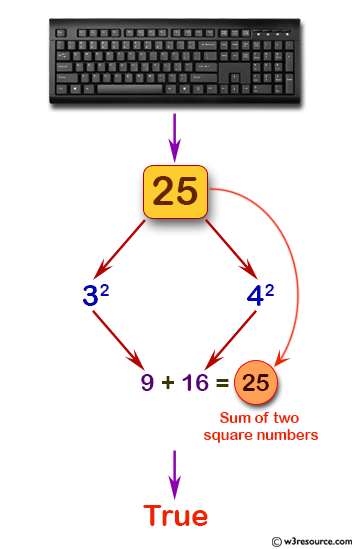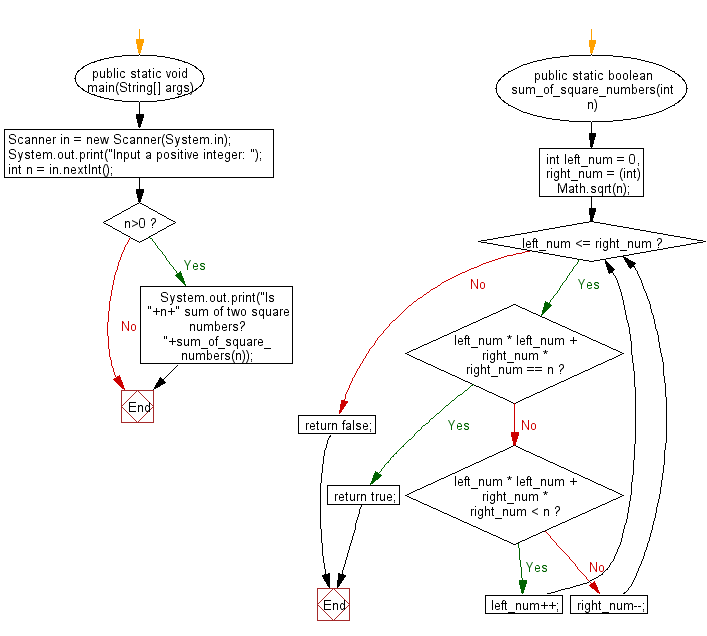﻿ Java programming exercises: Test whether there are two integers x and y - w3resource# Java Exercises: Test whether there are two integers x and y

## Java Basic: Exercise-191 with Solution

Write a Java program to test whether there are two integers x and y such that x^2 + y^2 is equal to a given positive number.

Pictorial Presentation:Sample Solution:

Java Code:

``````import java.util.*;
public class Solution {
public static void main(String[] args) {
Scanner in = new Scanner(System.in);
System.out.print("Input a positive integer: ");
int n = in.nextInt();
if (n>0)
{
System.out.print("Is "+n+" sum of two square numbers? "+sum_of_square_numbers(n));
}
}

public static boolean sum_of_square_numbers(int n) {
int left_num = 0, right_num = (int) Math.sqrt(n);
while (left_num <= right_num) {
if (left_num * left_num + right_num * right_num == n) {
return true;
} else if (left_num * left_num + right_num * right_num < n) {
left_num++;
} else {
right_num--;
}
}
return false;
}
}
```
```

Sample Output:

```Input a positive integer:  25
Is 25 sum of two square numbers? true
```

Flowchart:Java Code Editor:

Company:

What is the difficulty level of this exercise?

﻿

## Java: Tips of the Day

Array vs ArrayLists:

The main difference between these two is that an Array is of fixed size so once you have created an Array you cannot change it but the ArrayList is not of fixed size. You can create instances of ArrayLists without specifying its size. So if you create such instances of an ArrayList without specifying its size Java will create an instance of an ArrayList of default size.

Once an ArrayList is full it re-sizes itself. In fact, an ArrayList is internally supported by an array. So when an ArrayList is resized it will slow down its performance a bit as the contents of the old Array must be copied to a new Array.

At the same time, it's compulsory to specify the size of an Array directly or indirectly while creating it. And also Arrays can store both primitives and objects while ArrayLists only can store objects.

Ref: https://bit.ly/3o8L2KH